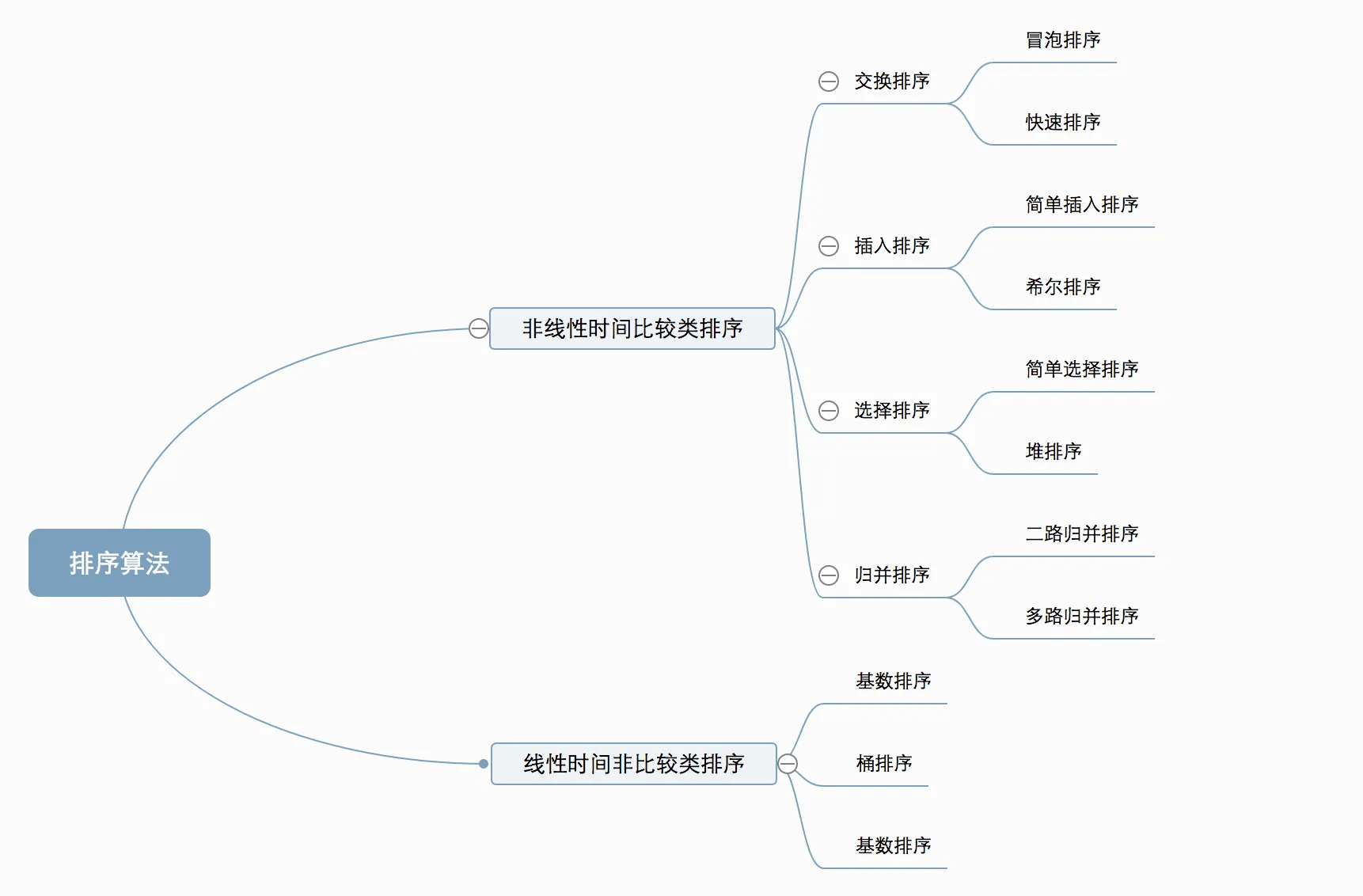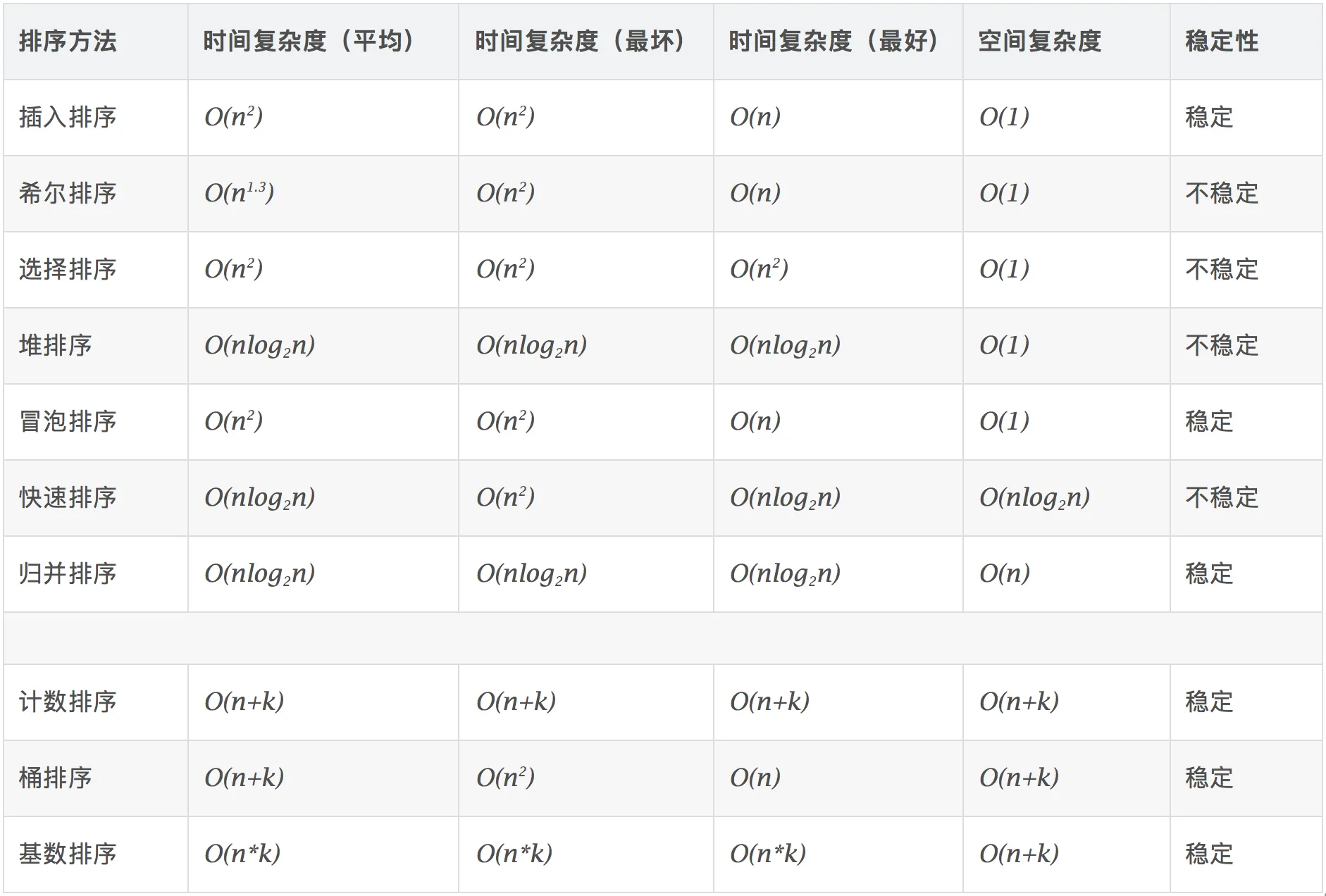## 1.冒泡排序(Bubble Sort)

### 1.2 动图演示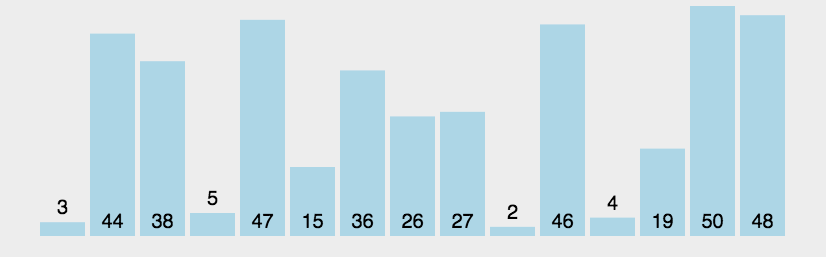### 1.3 Python3代码实现

    import numpy as np
import random

def bubble_sort(ownlist):
n = len(ownlist)

# Traverse through all array elements
for i in range(n):

# Last i elements are already in place
for j in range(0, n-i-1):

# traverse the array from 0 to n-i-1
# Swap if the element found is greater
# than the next element
if ownlist[j] > ownlist[j+1] :
ownlist[j], ownlist[j+1] = ownlist[j+1], ownlist[j]
print(ownlist)

a = random.sample(list(np.arange(100)),20)
bubble_sort(a)



## 2.选择排序(Selection Sort)

### 2.1 算法描述

n个记录的直接选择排序可经过n-1趟直接选择排序得到有序结果。

n-1次循环结束，数组变得有序化。

### 2.2 动图演示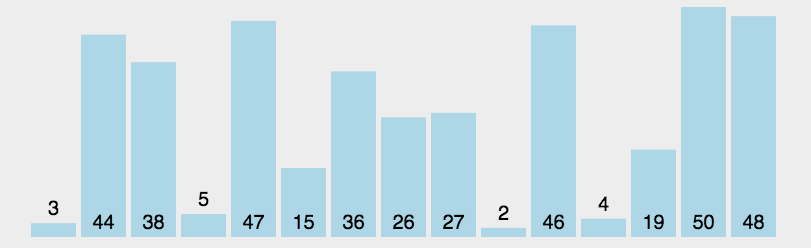### 2.3 Python3代码实现

    import numpy as np
import random

def selection_sort(ownlist):
n = len(ownlist)

for i in range(n):

minn = i
for j in range(i, n):

if ownlist[j] < ownlist[minn] :
minn = j
if minn!=i:
ownlist[i],ownlist[minn] = ownlist[minn],ownlist[i]
print(ownlist)

a = random.sample(list(np.arange(100)),20)
selection_sort(a)



## 3.插入排序(Insertion Sort)

### 3.1 算法描述

1.从第一个元素开始，该元素可以认为已经被排序；

2.取出下一个元素，在已经排序的元素序列中从后向前扫描；如果该元素（已排序）大于新元素，将该元素移到下一位置；重复直到找到已排序的元素小于或者等于新元素的位置；将新元素插入到该位置；

3重复步骤。

### 3.2 动图演示### 3.3 Python3代码实现

    import numpy as np
import random
def insertion_sort(ownlist):
for index in range(1,len(ownlist)):

currentvalue = ownlist[index]
position = index

while position>0 and ownlist[position-1]>currentvalue:
ownlist[position]=ownlist[position-1]
position = position-1

ownlist[position]=currentvalue
print(ownlist)
a = random.sample(list(np.arange(100)),20)
insertion_sort(a)



## 4.希尔排序(Shell Sort)

### 4.1 算法描述

• 选择一个增量序列t1，t2，…，tk，其中ti>tj，tk=1；
• 按增量序列个数k，对序列进行k 趟排序；
• 每趟排序，根据对应的增量ti，将待排序列分割成若干长度为m 的子序列，分别对各子表进行直接插入排序。仅增量因子为1 时，整个序列作为一个表来处理，表长度即为整个序列的长度。

### 4.2 动图演示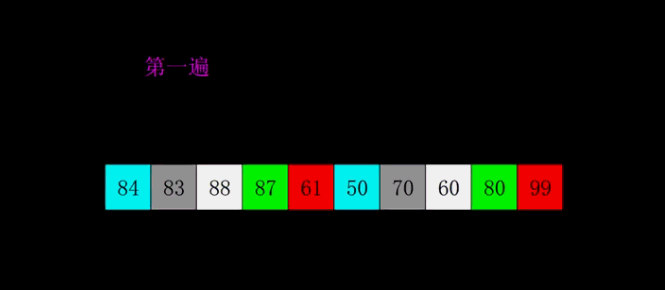### 4.3 Python3代码实现

    import numpy as np
import random
def shell_sort(ownlist):

n = len(ownlist)
gap = n//2
while gap > 0:

for i in range(gap,n):

temp = ownlist[i]

j = i
while  j >= gap and ownlist[j-gap] >temp:
ownlist[j] = ownlist[j-gap]
j -= gap

ownlist[j] = temp
gap //= 2
print(ownlist)
a = random.sample(list(np.arange(100)),20)
print(a)
shell_sort(a)



## 5.归并排序(Merge Sort)

### 5.2 动图演示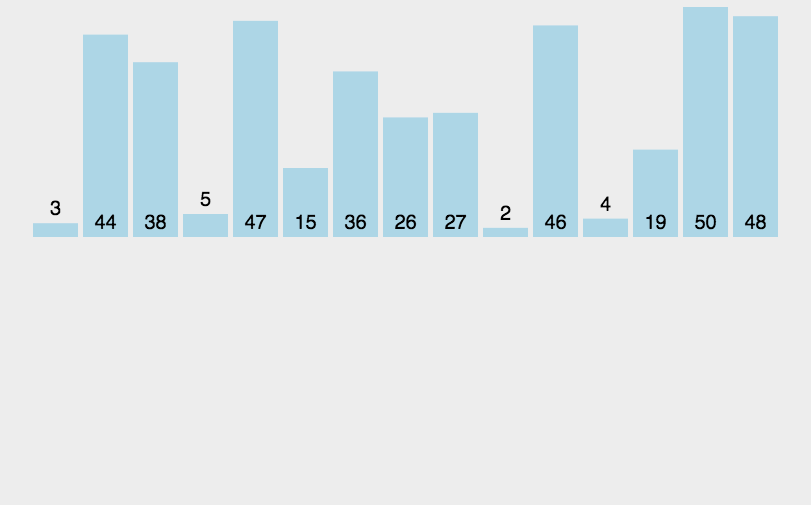### 5.3 Python3代码实现

    import numpy as np
import random
def merge_sort(ownlist):
print("分割 ",ownlist)
if len(ownlist)>1:
mid = len(ownlist)//2
lefthalf = ownlist[:mid]
righthalf = ownlist[mid:]

merge_sort(lefthalf)
merge_sort(righthalf)

i=0 #left
j=0  #right
k=0  #
while i < len(lefthalf) and j < len(righthalf):
if lefthalf[i] < righthalf[j]:
ownlist[k]=lefthalf[i]
i=i+1
else:
ownlist[k]=righthalf[j]
j=j+1
k=k+1

while i < len(lefthalf):
ownlist[k]=lefthalf[i]
i=i+1
k=k+1

while j < len(righthalf):
ownlist[k]=righthalf[j]
j=j+1
k=k+1
print("合并 ",ownlist)
a = random.sample(list(np.arange(100)),20)
print(a)
merge_sort(a)